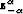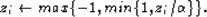Next: Least-squares solution of the Up: Algorithm for minimizing the Previous: An efficient algorithm for

# Minimizing the Huber function

So far I have introduced a general method for solving nonlinear problems. In this section I show how this algorithm can be used when the Huber function is utilized for measuring the data misfit. In fact, we only need to derive the gradient of the objective function in equation (). The gradient can be written in the following compact form Li and Swetist (1998):(84)
whereis a vector with ith component(85)
A last difficulty arises in the choice of the thresholdin equation (). This value remains constant during the iterations.is also the only parameter to choose a priori for different problems. I have not derived any analytical expression for, but based on previous works with IRLS methods Darche (1989), it seems that(86)
is a good practical choice. Another possible solution is to setat the 98-th percentile of the data (Claerbout, 2000, Personal communication).Next: Least-squares solution of the Up: Algorithm for minimizing the Previous: An efficient algorithm for
Stanford Exploration Project
5/5/2005